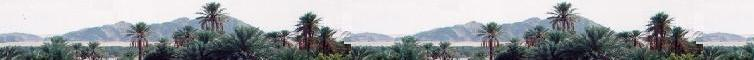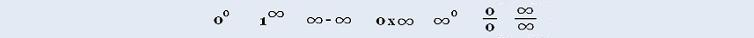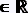###### Calculus I

Exercices

Applications

Marginal analysis

© The scientific sentence. 2010

# Calculus: Derivation rules

### 1. Derivative of a constant

The function f is constant . That is f(x) = b (b ∊ R)
so
lim (f(x + Δ x) - f(x))/Δx = (b - b)/Δx = 0
Δ x → 0

Rule 1:
f(x) constant
f'(x) = df(x)/dx = 0

### 2. Derivative of a power

The function f has the form:
f(x) = xn (n)

f'(x) = dxn/dx = lim (f(x + Δx) - f(x))/Δx
Δ x → 0
= lim ((x + Δx)n - xn)/Δx
Δ x → 0
0/0 : indetermined.

We have:
an - bn = (a - b)(an-1 + an-2b + an-3b2 + � + bn - 1 )

Therefore:

f'(x) = lim ((x + Δx)n - xn)/Δx
Δ x → 0
= lim ((x + Δx - x)((x+δx)n-1 + ... + xn-1)/Δx
Δ x → 0
= lim ((x + δx)n-1 + ... + xn-1)
Δ x → 0
(xn-1 + ... + xn-1)
= n xn-1

Rule 2:
f(x) = xn
f'(x) = n xn-1

### 3. Derivative of product of a constant by a function

The function f has the form:
f(x) = k g(x)
k.

f'(x) = lim (k g(x + Δx) - k g(x))/Δx
Δ x → 0
= k lim ( g(x + Δx) - g(x))/Δx
Δ x → 0
= k g'(x)

Rule 3:
f(x) = k g(x)
f'(x) = k g'(x)
k is a constant### 4. Derivative of the sum of two functions

The function f has the form:
f(x) = g(x) ± h(x)

f'(x) = lim (g(x + Δx) ± h(x + Δx) - g(x) - h(x))/Δx
Δ x → 0
= lim (g(x + Δx) - g(x))/Δx
Δ x → 0
± lim (h(x + Δx) - h(x))/Δx
Δ x → 0
= g'(x) ± h'(x)

Rule 4:
f(x) = g(x) ± h(x)
f'(x) = g'(x) ± h'(x)

The rule is generalized to more that two functions: [f(x) + g(x) + h(x) + ... + m(x)]' = f'(x) + g'(x) + h'(x) + ... + m'(x)

### 5. Derivative of the productof two functions

The function f has the form:
f(x) = g(x). h(x)

f'(x) = lim (g(x + Δx).h(x + Δx) - g(x).h(x))/Δx
Δ x → 0
= lim [g(x + Δx).h(x + Δx) - g(x).h(x) + g(x)h(x + Δx) - g(x)h(x + Δx) ]/Δx
Δ x → 0
= lim [(g(x + Δx) - g(x))h(x + Δx) + g(x)(h(x + Δx) - h(x)) ]/Δx
Δ x → 0
= lim [g'(x)h(x + Δx)] + g(x)h'(x)
Δ x → 0
= g'(x)h(x) + g(x)h'(x)

Rule 5:
f(x) = g(x).h(x)
f'(x) = g'(x)h(x) + g(x)h'(x)

### 6. Derivative of the division of two functions

The function f has the form:
f(x) = g(x)/h(x)

so
g(x) = f(x) h(x)

g'(x) = lim (g(x + Δx)h(x + Δx) - g(x)h(x))/Δx
Δ x → 0

Using the rule for the product of two functions, we have:

g'(x) = f'(x)h(x) + f(x)h'(x)

Therefore

g'(x) = f'(x)h(x) + f(x)h'(x)
[g'(x) - f(x)h'(x)]/h(x) = f'(x)

Since
g(x)/h(x) = f(x)

We have:
f'(x)= [h(x) g'(x) - g(x)h'(x)]/(h(x))2

Rule 6:
f(x) = g(x)/h(x)
f'(x)= [h(x) g'(x) - g(x)h'(x)]/(h(x))2

### 7. Derivative: Chain Rule

There is an usefull method to find a derivative of composite functions:

y = f(x) = g(h(x))

Let:
y = g(u) and
h(x) = u

We have:
Δy = g(h(x + Δx )) - g(h(x)), and
Δu = h(x + Δx) - h(x)

so

dy/dx = lim Δy/Δx
Δx → 0

Since

Δy/Δx = (Δy/Δu). (Δy/Δx), we get:

 dy/dx = lim (Δy/Δu) . Δ x → 0 lim (Δu/Δx) Δ x → 0

When Δx → 0
Δu = h(x + Δx) - h(x) → 0,

so:
 dy/dx = lim (Δy/Δu) . Δ u → 0 lim (Δu/Δx) Δ x → 0

= (dy/du) . (du/dx)

Rule 7: Chain Rule
f(x) = g(h(x))
y = g(u)
u = h(x)
f'(x)= (dy/du) . (du/dx)

### 8. Derivative of a power function

y = f(x) = (g(x))n,Let:
y = un and
g(x) = u

We have
dy/dx = (dy/du) . (du/dx)
= (nun-1) . (du/dx)
= (ng(x)n-1) . (dg(x)/dx)

Rule 8:
f(x) = (g(x))n
u = g(x)
f'(x) = dy/dx = n(g(x))n-1. (dg(x)/dx)

### 9. Exercices:

 chimie labs | Physics and Measurements | Probability & Statistics | Combinatorics - Probability | Chimie | Optics | contact |